# Nesting

Nested functions are just functions within functions. The result returned from one function is used as the argument to another function.
Your calculations will often involve using several worksheet functions in order to calculate the desired result.
A simple example might be obtaining the first name from a cell entry that contains both a first name and a surname.
In this simple example we use the following two functions:
1) The FIND function to locate the character position of the first space in the text "Russell Proctor".
2) The LEFT function to obtain all the text that is to the left of this space, to return the first word "Russell".
Cell "C2" contains a persons full name and we want to create a formula that will return just their first name.
This could be done with two separate formulas. The first formula in cell "C4" obtains the position of the space character.
The second formula in cell "C5" returns all the characters that are to the left of the character containing the space.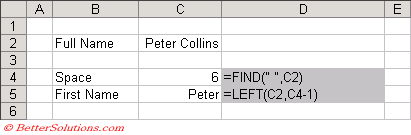The value returned from the formula in "C5" is the correct answer, in this case Peter.
To make your formulas more efficient and to reduce the number of cells needed it is possible combine the two functions FIND() and LEFT() into a single formula. This creates a formula containing a nested function.
The formula in cell "C4" now contains both the functions. The FIND() function has been nested inside the LEFT() function.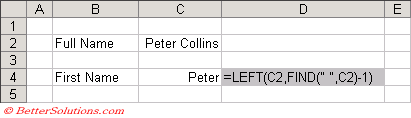The value returned from the formula in "C4" is the correct answer and uses a more concise formula.

### Entering Nested Functions

When you enter a formula you can type the name of a function directly into the formula bar.
If you do not know the name of the function then you can either press the "Insert Function" button on the formula bar or you can select (Insert > Function).
You can alternatively insert a function by using the drop-down box that has replaced the Name Box and either select the function from the list or select "More Functions" (at the bottom) to display the "Insert Function" dialog box.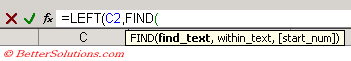You can nest up to seven functions within the same formula.
When nesting functions you should try to use extra parentheses where necessary in order to make the formula as intuitive as possible.

### Nested IF functions

Probably the most common use of nested functions is to perform conditional tests.
Nested IF functions are a common conditional test although being limited to seven nested functions can cause problems.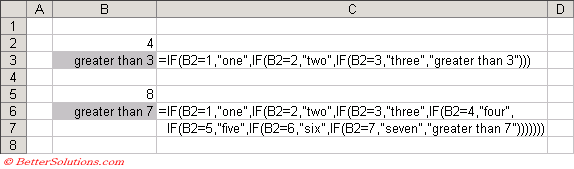If you want to use more than seven functions then you will have to break up the formula into smaller formulas.

### Nesting other functions

You can nest any types of functions as long as the arguments are of the correct datatype.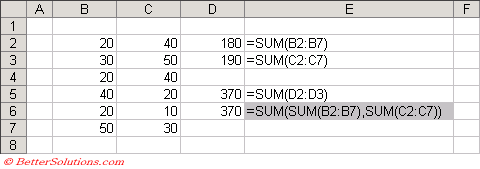When you insert cells at the bottom or to the right of a range referenced by a formula, the formulas will be automatically adjusted for you as soon as you type values into the new inserted cells.
This is known as "Automatic Range Expansion" and only works when you insert cells immediately to the right or below a referenced range.

### Important

You cannot nest more than seven worksheet functions in a single formula.
If a formula contains several functions (maybe nested) you can change which function is displayed in the Function Arguments dialog box by simply clicking on the function name in the formula bar.
You can display all the function arguments by pressing (Ctrl + A) when the insertion point is to the right of a function name in a formula.